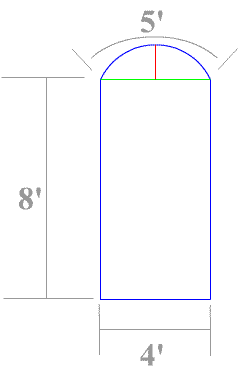Temporary Work _______ 20060514 From the Arched Door math problem from page 21 of Barnett and Ziegler We are presented with a problem where the cord can be found (4/5=1.25). On Constructor’s Corner we have already found 4 different ways to solve this problem. However this solution is simple and proves to be quite interesting. Here is a simple, little theory that this solution is based on: A chord will make a circle with a radius equal to the chord. But imagine if the chord increased in length as it rotated around the circle. This increase in length adding to the chord so that the chord now draws the circle that encompasses it. It just so happens that the cord increases “inversely” to maintain the same arc length as the original radius. In the Arched Doorway problem we already know that at 180 degrees along the horizontal line, the chord equals 2. (We would have to know an angle if the angle wasn’t perpendicular.) So 1/(1.25/2) = 2 the circle increases by so much of a percent per so many degrees So the percentage increase doubles by 90 degrees; so the length of movement is 4 that is the horizontal distance 1 direction from 90 to 180 and then from 180 to 270 1\(1.25\4) = 3.2 + 1.25 = 4.45 This number is the diameter. (3.2 + 1.25)\2 = 2.225 This number is the radius.